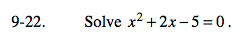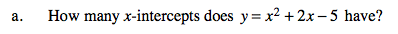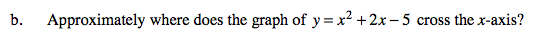### Home > A2C > Chapter 9 > Lesson 9.1.1 > Problem9-22

9-22.
1. Solve x2 + 2x − 5 = 0. Homework Help ✎

1. How many x-intercepts does y = x2 + 2x − 5 have?

2. Approximately where does the graph of y = x2 + 2x − 5 cross the x-axis?How many solutions did you find?x ≈ 1.45 and x ≈ −3.45